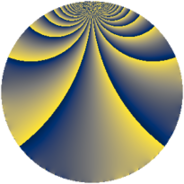# Properties

 Label 3872.1.f.bLevel $3872$ Weight $1$ Character orbit 3872.f Self dual yes Analytic conductor $1.932$ Analytic rank $0$ Dimension $2$ Projective image $D_{5}$ CM discriminant -8 Inner twists $2$

# Related objects

## Newspace parameters

 Level: $$N$$ $$=$$ $$3872 = 2^{5} \cdot 11^{2}$$ Weight: $$k$$ $$=$$ $$1$$ Character orbit: $$[\chi]$$ $$=$$ 3872.f (of order $$2$$, degree $$1$$, not minimal)

## Newform invariants

 Self dual: yes Analytic conductor: $$1.93237972891$$ Analytic rank: $$0$$ Dimension: $$2$$ Coefficient field: $$\Q(\sqrt{5})$$ Defining polynomial: $$x^{2} - x - 1$$ Coefficient ring: $$\Z[a_1, a_2, a_3]$$ Coefficient ring index: $$1$$ Twist minimal: no (minimal twist has level 88) Projective image: $$D_{5}$$ Projective field: Galois closure of 5.1.937024.1

## $q$-expansion

Coefficients of the $$q$$-expansion are expressed in terms of $$\beta = \frac{1}{2}(1 + \sqrt{5})$$. We also show the integral $$q$$-expansion of the trace form.

 $$f(q)$$ $$=$$ $$q + \beta q^{3} + \beta q^{9} +O(q^{10})$$ $$q + \beta q^{3} + \beta q^{9} + ( 1 - \beta ) q^{17} + ( -1 + \beta ) q^{19} + q^{25} + q^{27} + \beta q^{41} -\beta q^{43} + q^{49} - q^{51} + q^{57} + ( 1 - \beta ) q^{59} + ( 1 - \beta ) q^{67} + \beta q^{73} + \beta q^{75} -\beta q^{83} -\beta q^{89} -\beta q^{97} +O(q^{100})$$ $$\operatorname{Tr}(f)(q)$$ $$=$$ $$2q + q^{3} + q^{9} + O(q^{10})$$ $$2q + q^{3} + q^{9} + q^{17} - q^{19} + 2q^{25} + 2q^{27} + q^{41} - q^{43} + 2q^{49} - 2q^{51} + 2q^{57} + q^{59} + q^{67} + q^{73} + q^{75} - q^{83} - q^{89} - q^{97} + O(q^{100})$$

## Character values

We give the values of $$\chi$$ on generators for $$\left(\mathbb{Z}/3872\mathbb{Z}\right)^\times$$.

 $$n$$ $$485$$ $$1695$$ $$2785$$ $$\chi(n)$$ $$-1$$ $$-1$$ $$1$$

## Embeddings

For each embedding $$\iota_m$$ of the coefficient field, the values $$\iota_m(a_n)$$ are shown below.

For more information on an embedded modular form you can click on its label.

Label $$\iota_m(\nu)$$ $$a_{2}$$ $$a_{3}$$ $$a_{4}$$ $$a_{5}$$ $$a_{6}$$ $$a_{7}$$ $$a_{8}$$ $$a_{9}$$ $$a_{10}$$
3631.1
 −0.618034 1.61803
0 −0.618034 0 0 0 0 0 −0.618034 0
3631.2 0 1.61803 0 0 0 0 0 1.61803 0
 $$n$$: e.g. 2-40 or 990-1000 Significant digits: Format: Complex embeddings Normalized embeddings Satake parameters Satake angles

## Inner twists

Char Parity Ord Mult Type
1.a even 1 1 trivial
8.d odd 2 1 CM by $$\Q(\sqrt{-2})$$

## Twists

By twisting character orbit
Char Parity Ord Mult Type Twist Min Dim
1.a even 1 1 trivial 3872.1.f.b 2
4.b odd 2 1 968.1.f.a 2
8.b even 2 1 968.1.f.a 2
8.d odd 2 1 CM 3872.1.f.b 2
11.b odd 2 1 3872.1.f.a 2
11.c even 5 2 3872.1.t.a 4
11.c even 5 2 3872.1.t.c 4
11.d odd 10 2 352.1.t.a 4
11.d odd 10 2 3872.1.t.b 4
33.f even 10 2 3168.1.ck.a 4
44.c even 2 1 968.1.f.b 2
44.g even 10 2 88.1.l.a 4
44.g even 10 2 968.1.l.a 4
44.h odd 10 2 968.1.l.b 4
44.h odd 10 2 968.1.l.c 4
88.b odd 2 1 968.1.f.b 2
88.g even 2 1 3872.1.f.a 2
88.k even 10 2 352.1.t.a 4
88.k even 10 2 3872.1.t.b 4
88.l odd 10 2 3872.1.t.a 4
88.l odd 10 2 3872.1.t.c 4
88.o even 10 2 968.1.l.b 4
88.o even 10 2 968.1.l.c 4
88.p odd 10 2 88.1.l.a 4
88.p odd 10 2 968.1.l.a 4
132.n odd 10 2 792.1.bu.a 4
176.u odd 20 4 2816.1.v.c 8
176.x even 20 4 2816.1.v.c 8
220.o even 10 2 2200.1.cl.a 4
220.w odd 20 4 2200.1.dd.a 8
264.r odd 10 2 3168.1.ck.a 4
264.u even 10 2 792.1.bu.a 4
440.ba odd 10 2 2200.1.cl.a 4
440.bu even 20 4 2200.1.dd.a 8

By twisted newform orbit
Twist Min Dim Char Parity Ord Mult Type
88.1.l.a 4 44.g even 10 2
88.1.l.a 4 88.p odd 10 2
352.1.t.a 4 11.d odd 10 2
352.1.t.a 4 88.k even 10 2
792.1.bu.a 4 132.n odd 10 2
792.1.bu.a 4 264.u even 10 2
968.1.f.a 2 4.b odd 2 1
968.1.f.a 2 8.b even 2 1
968.1.f.b 2 44.c even 2 1
968.1.f.b 2 88.b odd 2 1
968.1.l.a 4 44.g even 10 2
968.1.l.a 4 88.p odd 10 2
968.1.l.b 4 44.h odd 10 2
968.1.l.b 4 88.o even 10 2
968.1.l.c 4 44.h odd 10 2
968.1.l.c 4 88.o even 10 2
2200.1.cl.a 4 220.o even 10 2
2200.1.cl.a 4 440.ba odd 10 2
2200.1.dd.a 8 220.w odd 20 4
2200.1.dd.a 8 440.bu even 20 4
2816.1.v.c 8 176.u odd 20 4
2816.1.v.c 8 176.x even 20 4
3168.1.ck.a 4 33.f even 10 2
3168.1.ck.a 4 264.r odd 10 2
3872.1.f.a 2 11.b odd 2 1
3872.1.f.a 2 88.g even 2 1
3872.1.f.b 2 1.a even 1 1 trivial
3872.1.f.b 2 8.d odd 2 1 CM
3872.1.t.a 4 11.c even 5 2
3872.1.t.a 4 88.l odd 10 2
3872.1.t.b 4 11.d odd 10 2
3872.1.t.b 4 88.k even 10 2
3872.1.t.c 4 11.c even 5 2
3872.1.t.c 4 88.l odd 10 2

## Hecke kernels

This newform subspace can be constructed as the kernel of the linear operator $$T_{17}^{2} - T_{17} - 1$$ acting on $$S_{1}^{\mathrm{new}}(3872, [\chi])$$.

## Hecke characteristic polynomials

$p$ $F_p(T)$
$2$ $$T^{2}$$
$3$ $$-1 - T + T^{2}$$
$5$ $$T^{2}$$
$7$ $$T^{2}$$
$11$ $$T^{2}$$
$13$ $$T^{2}$$
$17$ $$-1 - T + T^{2}$$
$19$ $$-1 + T + T^{2}$$
$23$ $$T^{2}$$
$29$ $$T^{2}$$
$31$ $$T^{2}$$
$37$ $$T^{2}$$
$41$ $$-1 - T + T^{2}$$
$43$ $$-1 + T + T^{2}$$
$47$ $$T^{2}$$
$53$ $$T^{2}$$
$59$ $$-1 - T + T^{2}$$
$61$ $$T^{2}$$
$67$ $$-1 - T + T^{2}$$
$71$ $$T^{2}$$
$73$ $$-1 - T + T^{2}$$
$79$ $$T^{2}$$
$83$ $$-1 + T + T^{2}$$
$89$ $$-1 + T + T^{2}$$
$97$ $$-1 + T + T^{2}$$